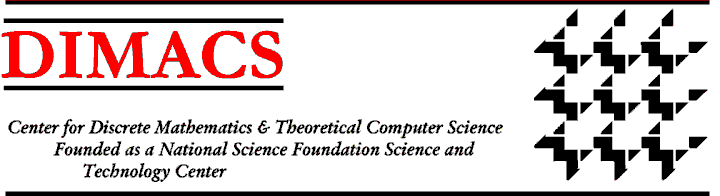### DIMACS/CCICADA Workshop on Risk-Averse Algorithmic Decision Making

#### May 9 - 11, 2011 DIMACS Center, CoRE Building, Rutgers University

Organizers:
Melike Baykal-Gursoy, Rutgers University, gursoy at rci.rutgers.edu
David Brown, Duke University, dbbrown at duke.edu
Aleksandar Pekec, Duke University, pekec at duke.edu
Andrzej Ruszczynski, Rutgers University, rusz at business.rutgers.edu
Dharmashankar Subramanian, IBM Watson Labs dharmash at us.ibm.com
Presented under the auspices of the Special Focus on Algorithmic Decision Theory and The Homeland Security Center for Command, Control, and Interoperability Center for Advanced Data Analysis (CCICADA).

Properly assessing riskiness of available options is central to decision making under uncertainty. Measures of risk are often important in the context of solving dynamic optimization problems. This workshop will concentrate on recent work developing alternative measures and their properties, especially in the context of dynamic optimization. Since a risk measure has to be efficiently computed to be useful, there is a need to develop efficient algorithms that address dynamic risk measures in both the objective function and the constraints. This calls for new methods of ADT. It also calls for new methods for modeling constraints in a dynamic setting in a practically useful manner and for ways to understand the interplay among risk measures, problem formulation, and computational tractability.

Standard risk measures are sometimes captured by the second moment of multidimensional random variables (i.e., the covariance matrix). However, these are often not the most appropriate for a problem at hand. For example, Value-at-Risk ($VaR$) is a prevalent measure used by financial institutions: It measures one's exposure to large losses. (More precisely, for a random variable $X$, VaR at the confidence level $\alpha$, $VaR_\alpha(X)$, is defined as the value $P(X < VaR_\alpha(X))=1-\alpha$, i.e., there is only a $1-\alpha$ chance for $X$ to attain a value below $VaR$.). The improper use of $VaR$ in risk management has been pinpointed as one of the critical factors that led to the financial crisis of 2008 and its role in the crisis has even been subject to a Congressional hearing. Computing $VaR$ across the portfolio of investments is a well-studied optimization problem, but many logical improvements and extensions of $VaR$, such as Conditional Value-at-Risk ($CVaR$) that measures expected value of the loss given the loss is larger than some threshold, pose interesting and challenging optimization issues that we will explore.

Efficient algorithms have been developed for solving single-stage, static mathematical programs that involve a CVaR risk measure in the objective function and/or constraints. However, extending the ideas to address risk-aware optimization problems in a dynamic setting is a relatively new area. Such extension involves choice of an appropriate dynamic risk measure that satisfies the notion of time-consistency. A characterization for such measures in the form of conditional risk mappings can be found in. Other papers address incorporating such risk measures in the objective function for risk-averse 2-stage linear programs and Markov decision problems.

Recent research efforts have focused on the interplay between decision making with classes of risk measures and robust and stochastic optimization. For example, uncertainty sets in the context of robust optimization formulations are related to risk measures proposes a more general model of robust optimization and applies the approach to stochastic optimization problems involving ambiguity in the underlying probability distribution; this approach is shown to be closely connected to convex risk measures developed. Recent work developing models of choice involving aspiration levels as well as approaches for performance evaluation have also drawn on dual connections to risk measures obeying desirable properties. Thus, there is a further need to develop optimization techniques and algorithms for computing risk measures, and to understand the limitations that existing optimization algorithms impose on the viability of a risk measure when efficiency in computing is important. Consequently, the WS will also focus on practically useful modeling techniques and efficient algorithmic approaches for incorporating risk measures in the constraints and/or objective function.Next: Call for ParticipationWorkshop IndexDIMACS Homepage
Contacting the Center